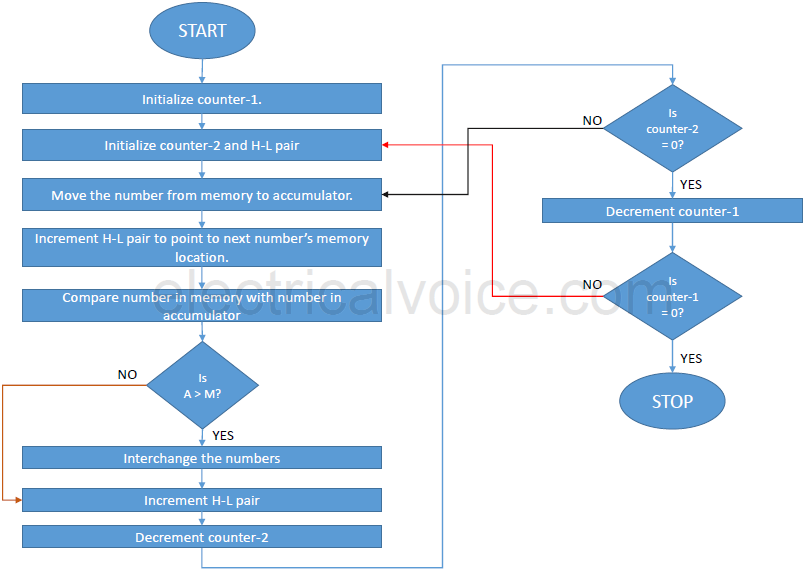# Program to Sort the array in Ascending Order

Q. Write an 8085 program and draw a flowchart to Sort the array in Ascending Order.(8085 Microprocessor Program)

Flowchart/AlgorithmProgram

 Address Mnemonics Operand Opcode Comments 2000 MVI B, 05H 06 Initialize counter-1. 2001 05 2002 MVI C, 05H 0E Initialize counter-2. 2003 05 2004 LXI H, 3000H 21 Load H-L pair with address 3000H. 2005 00 2006 30 2007 MOV A, M 7E Move the number from memory to reg. A. 2008 INX H 23 Increment H-L pair. 2009 CMP M BD Compare the number with next number. 200A JC 2015H DA Don’t interchange if number < next number. 200B 15 200C 20 200D JZ 2015H CA Don’t interchange if number = next number. 200E 15 200F 20 2010 MOV D, M 56 Otherwise, swap the numbers. Move next number from memory to D. 2011 MOV M, A 77 Move the first number from A to memory. 2012 DCX H 2B Decrement H-L pair. 2013 MOV M, D 72 Move next number from D to memory. 2014 INX H 23 Increment H-L pair. 2015 DCR C 0D Decrement counter 2. 2016 JNZ 2007H C2 If counter-2 ≠ 0, repeat. 2017 07 2018 20 2019 DCR B 05 Decrement counter-1. 201A JNZ 2002 C2 If counter-1 ≠ 0, repeat. 201B 02 201C 20 201D HLT 76 Halt.

Output

Before Execution:

3000H: 05H
3001H: 15H
3002H: 01H
3003H: 65H
3004H: 32H

After Execution:

3000H: 01H
3001H: 05H
3002H: 15H
3003H: 32H
3004H: 65H

Program Explanation

1. This program sorts an array in ascending order.
2. Let us assume that there are five numbers in the array and its starting address is 3000H.
3. Initially, counter-1 and counter-2 are initialized with the size of the array.
4. H-L pair is pointed to the starting address of the array.
5. In the first iteration, the first number is compared with the second number.
6. If the first number < second number, then do not interchange them. Otherwise, if the first number > second number, then swap them.
7. In the next iteration, the first number is compared with the third number.
8. If first number < third number, then do not interchange them. Otherwise, if first number > third number, then swap them.
9. In the next iteration, the first number is compared with the fourth number and the process continues until counter-2 becomes zero.
10. When counter-2 becomes zero, counter-1 is decremented and the process continues until all the numbers are arranged in ascending order.
error: Content is protected !!